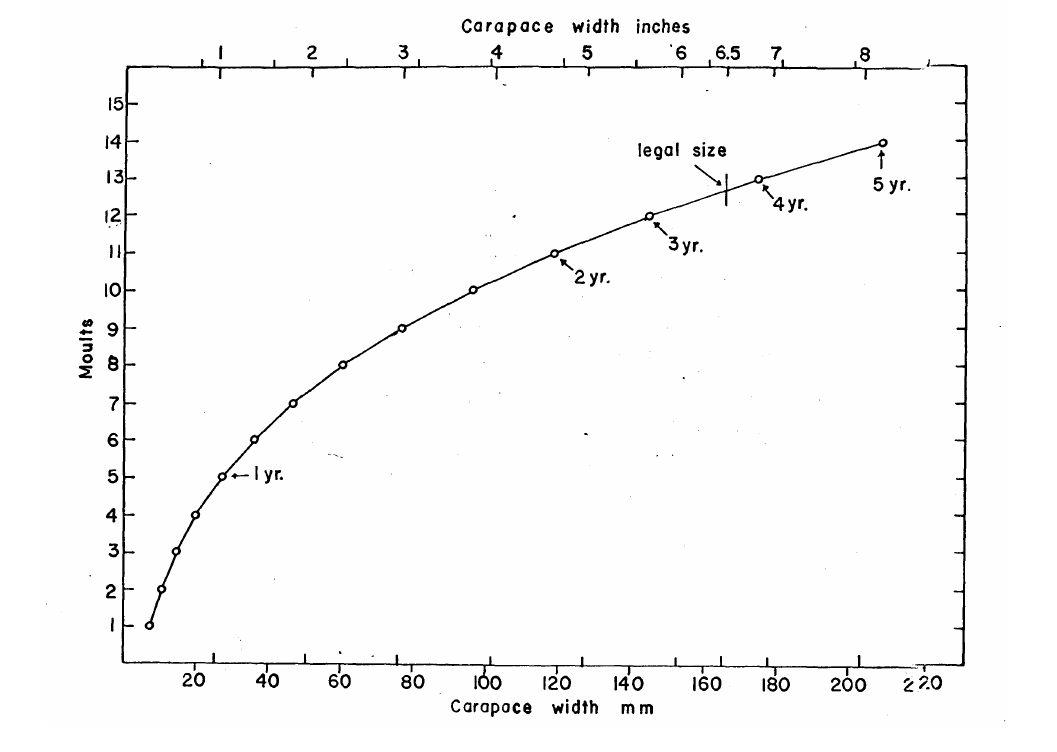### Evaluating rules for opening and closing the crab fishery

1. Investigation of weekly exploitation rates
2. Evaluation of possible seasonal changes in crab vulnerability
3. Prospective simulation

### Three Chapters

1. Introduce model and fit to data assuming only size dependent vulnerability.
2. Extend model to the time dependent vulnerability regimes and use size composition data from soft shell survey.
3. Prospective analysis

### Chapter 1 Model

1. Fit model to weekly catch and effort data
2. Develop main components of model
3. Simulation test model

### Flow chart of Population Model|Shell Condition|Number of Weeks| |------|:---| |1|6| |2|4| |3|6| |4|2| |5|1| |6|8| |8|25| |7|1000|
|Shell Condition|Weekly Mortality| |------|:---| |1|.0001\$$\mu\$$| |2|.002\$$\mu\$$| |3|.003\$$\mu\$$| |4|.005\$$\mu\$$| |5|.01\$$\mu\$$| |6|.001\$$\mu\$$| |8|.001\$$\mu\$$| |7|.1\$$\mu\$$|

### Mortality

$$A=A(1-M_{sc})$$

### Negative Log-Likelihood

$$nll(x) = \sum{-log(\frac{1}{{\sigma \sqrt {2\pi } }}e^{{{ - \frac{\left( {C - \hat{C} } \right)^2}{2 \sigma^2} }}}})$$ With the right indices to make catches by week make sense.

### Chapter 1 Results

1. Are there seasonal patterns in weekly exploitation rates?
2. Is there evidence, in the depletion series, for the commonly held belief that more than $$90 \%$$ of the legal crabs are harvested?$$cv=.05$$### Chapter 2 Vulnerability Regime

2. Able to estimate a scalar natural mortality
3. Likelihood profiles of various vulnerability regimes

### Time-Dependent Vulnerability Hypothesis

1. Crabs feed more intensely just prior to and after hardening up after a molt and make themselves more vulnerable to capture during these shell conditions
2. There are simply seasonal changes in vulnerability, these changes do not depend on shell condition or number of traps in the water.
3. Crabs aggregate due to the presence of baited traps, increasing catch rate

### Shell condition vulnerability

The Catch equation becomes $$C=qv_{a,t}E_tA_t$$ \begin{eqnarray} v_{a,t}&=&1 \mbox{ if } SC_a=1 \\ v_{a,t}&=&\alpha \mbox{ if } SC_a\ne 1 \end{eqnarray}

### Seasonal Vulnerability

The Catch equation becomes $$C=qv_{a,t}E_tA_t$$

Crabs are fully vulnerable from May until September.

These dates are arbitrary. \begin{eqnarray} v_{a,t}&=&1 \mbox{ if } 18\leq t \leq36 \\ v_{a,t}&=&\alpha \mbox{ otherwise } \end{eqnarray} Shortened period of full vulnerability \begin{eqnarray} v_{a,t}&=&1 \mbox{ if } 22\leq t \leq26 \\ v_{a,t}&=&\alpha \mbox{ otherwise } \end{eqnarray}

### Effort Vulnerability

\begin{eqnarray} \label{eq:effortvul} VW_{t+1}&=&.4\frac{E_t}{40000}+(1-.4)VW_t\\ v_{a,t}&=&1 \mbox{ if } \alpha\cdot VW_t >=1 \mbox{ or } SC_a=1 \mbox{ and } weeks<=2\\ v_{a,t}&=&\alpha VW_t \end{eqnarray}

### Time-Independent Vulnerability

1. Vulnerability varies randomly between crabs
2. Vulnerability is constant between all crabs.

### Variable vulnerability

$$V_{a}\sim U(0,1)$$

### Negative Log-Likelihood

$$nll(x) = \sum{-log(\frac{1}{{\sigma \sqrt {2\pi } }}e^{{{ - \frac{\left( {C - \hat{C} } \right)^2}{2 \sigma^2} }}}})-\sum{S \cdot log(\hat{S}) W_{sample} }$$ With the right indices to make proportions at size for each year and catches by week make sense.

### Chapter 2 Results

1. How to figure out quantitative vulnerability scenarios?
2. What drives effort?
3. What Management Scenarios and indicators am I missing?
Seasonal NLLSeasonal Short NLLShell NLLEffort NLL### $$CVRMSE$$

|sim|est|q|TC|OC|A|U| |:---|---|---|---|---|---|---|---|---|---|---|---|---| |const|const | -0.00 | -0.05 | 0.02 | 0.05 | 0.04| |const|var | 1.63 | 0.40 | 1.05 | 0.05 | 2.59 | |const|shell | -0.35 | 1.18 | 0.30 | 0.04 | 0.59| |var| const | -0.44 | -0.27 | 0.28 | 0.03 | 0.61| |var|var | 0.00 | -0.07 | 0.02 | 0.03 | 0.03| |var|shell | -0.36 | 0.67 | 0.33 | 0.03 | 0.46 | |shell|const | 0.46 | 0.08 | 0.82 | 0.04 | 0.63| |shell|var |0.62 | 0.15 | 0.59 | 0.04 | 1.42 | |shell|shell |0.00 |-0.06 | 0.08 | 0.04 | 0.09|

### Chapter 3

1. Three different rules for setting opening and closing dates
2. Four different vulnerability hypothesis: constant, variable, shell condition and effort.
3. Two different models of effort: a linear function of cpue in the previous week and a linear function of cpue in the previous week and average windspeed in present week.
\begin{eqnarray} Effort_{t+1}&=& \beta (catch_t)^\alpha\\ \alpha&=&0.54\\ \beta&=&1.59\\ \end{eqnarray}
Effort and CPUE